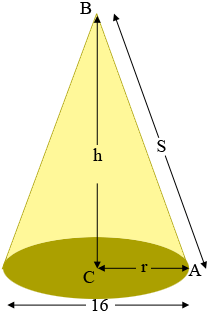SEARCH HOMEMath Central Quandaries & QueriesQuestion from Edwin: In making a 16" dia. cone lamp (stained glass), how many square feet of glass do I need.Hi Edwin,

I need to know how tall you want the lamp. I need either the height $h$ or the slant height $s.$Penny

We received the reply : h- six &one half” S- 9”

In my digram $ABC$ is a right triangle so Pythagoras Theorem gives

$S^2 = r^2 + h^2$

and thus if $r = 8$ inches and $h = 6$ inches than

$S^2 = 8^2 + 6.5^2 = 106.25$

and thus $S = 10.3$ inches so I am not sure what value to use for $S.$

Regardless, if you look at Stephen's response to a similar question you will find the expression

$\frac{\mbox{lateral area}}{\pi \; S^2} = \frac{2 \pi \; r}{2 \pi \; S}$

which simplifies to

$\mbox{lateral area} = \pi \; r \times S.$

I hope this helps,
PennyMath Central is supported by the University of Regina and the Imperial Oil Foundation.